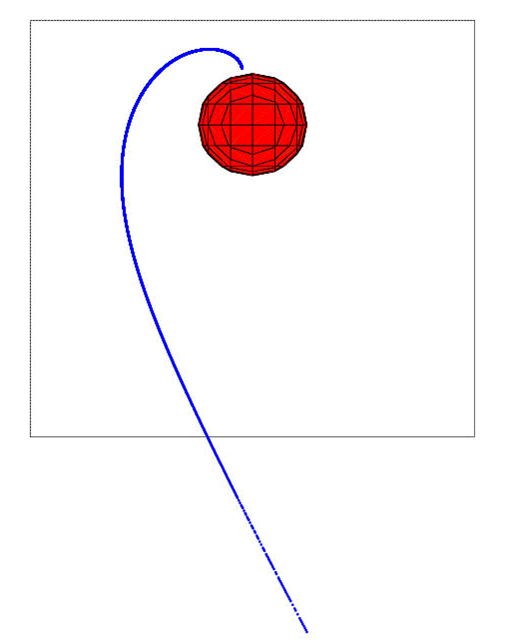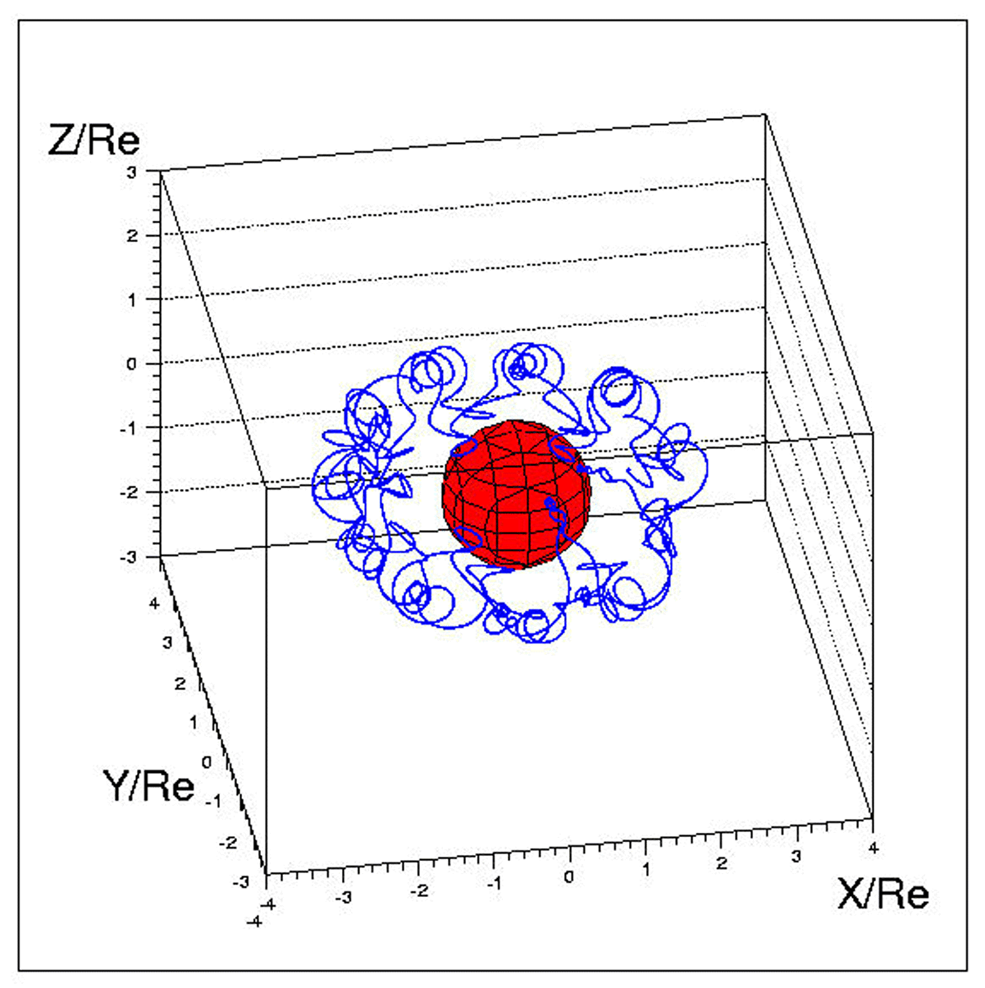Before starting the reconstruction of the trajectory, the existence of the external parameters like the solar wind ram pressure $$p_{dyn}$$, Dst-index and transverse components (B$$_y$$ and B$$_z$$) of the interplanetary magnetic field at each given date are checked. If these parameters are available, they must to be also inside the following limits: $$p_{dyn}$$ between 0.5 and 10 nPa, Dst-index between -100 and 20 nT, IMF-B$$_y$$ and IMF-B$$_z$$ between -10 and 10 nT. For the TS05 models other six parameters are required; ($$W_1,...,W_6$$) are functions of solar wind density $$N_{sw}$$, speed $$V_{sw}$$ and the southward interplanetary magnetic field B$$_z$$. After the checks on the parameters it is possible to start the reconstruction of the particle trajectory back in time.